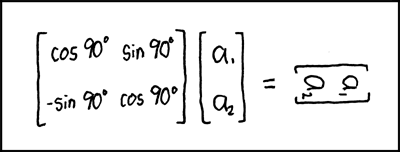# Difference between revisions of "184: Matrix Transform"

 Matrix TransformTitle text: In fact, draw all your rotational matrices sideways. Your professors will love it! And then they'll go home and shrink.

## Explanation

A rotational matrix transformation (i.e. the big brackets with a few "cos" and "sin" in them) is used in computer graphics to rotate an image. The product of the transform matrix and the argument vector (a1, a2) is a rotated version of the argument vector, which should be noted as (a1', a2').

The joke is that the author turned the image of the vector rather than writing the correct answer. Rotational matrix transformations are a special case of the general linear matrix transform, which can do other things to images, including shrinking them. In this case the matrix would rotate the image 90 degrees clockwise.

At the title text we see that the professors even shrinking at home on this joke.

## Transcript

[A square matrix next to a vertical two-by-one matrix, equated to a horizontal matrix that looks like the two-by-one matrix turned 90 degrees.]
[Square matrix:
cos90° sin90°
-sin90° cos90°]
[Two by one matrix:
a₁
a₂]
[An equal sign]
[The same two by one matrix, but rotated by 90 degrees clockwise:
a₁
a₂]add a comment! ⋅add a topic (use sparingly)! ⋅refresh comments!

# Discussion

This baby needs a bit more rigor. --Quicksilver (talk) 05:23, 24 August 2013 (UTC) 173.245.62.84 07:54, 7 April 2014 (UTC) I think this is also a reference to the movie "The Matrix", specifically the now famous scene where Neo does 90degree back bending to dodge bullets. 173.245.62.84 07:54, 7 April 2014 (UTC)

Maybe another reference: They'll go home (translation matrix) and shrink (scale matrix). Translation, scale and rotate are probably the most popular linear transformations. 108.162.218.89 23:39, 14 May 2014 (UTC)

I've been teach that the rotation was anticlockwise. Do computer turn the other way round that math teachers? by the way (left ( matrix{0 # 1 ## -1 # 0} right )*left ( binom 1 0 right)=left (binom 0 1 right) if you know what i mean. get fun. Yomismo (talk) 09:28, 18 March 2015 (UTC)

Iiiii'm pretty sure the "shrink" is a typo, and he meant "drink". My evidence is the xkcd book, where he just flat-out says it.

On clockwise/counterclockwise: I think Randall is using screen coordinates (where the top left is (0,0) and the point (1,4) is one below (1,3)), instead of standard Cartesian coordinates where the bottom left is (0,0) and going from (1,3) to (1,4) means going upwards. 162.158.155.170 13:07, 17 May 2019 (UTC)